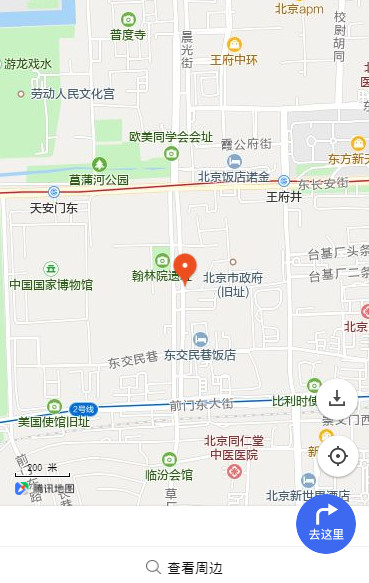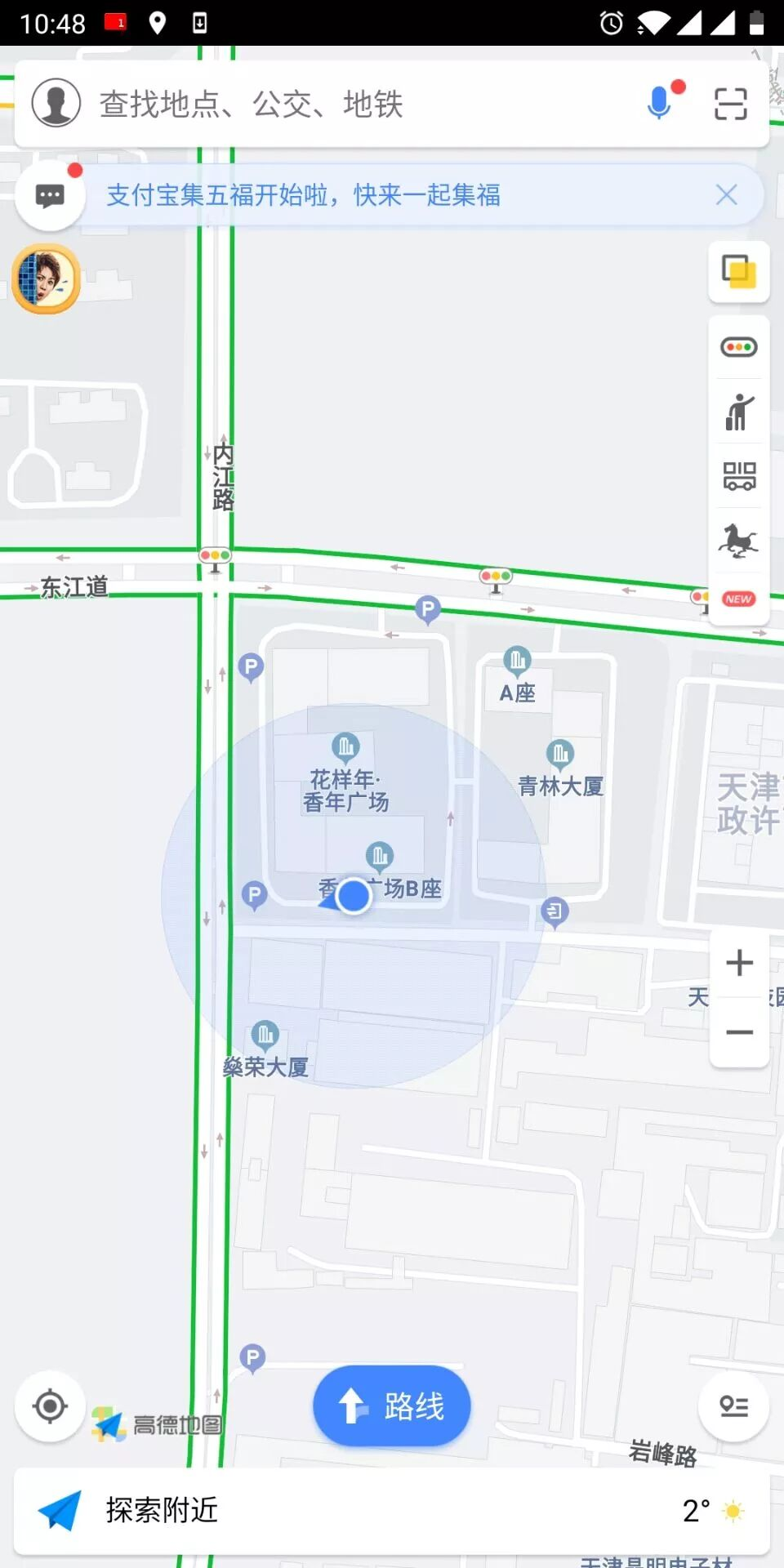# 置顶获取当前位置坐标问题精选热门

- 当前 Bug 的表现（可附上截图）

latitude=39.90469;longitude=116.40717;speed=-1;accuracy=65；显示如上图所示。`getBiddingList: ``function` `() {``    ``var` `that = ``this``;``    ``wx.getLocation({``      ``type:``'wgs84'``,``      ``success: ``function``(res) {``        ``const` `latitude = res.latitude``        ``const` `longitude = res.longitude``        ``const` `speed = res.speed``        ``const` `accuracy = res.accuracy``        ``console.log(``'latitude='` `+ latitude + ``';longitude='` `+ longitude + ``';speed='` `+ speed + ``';accuracy='` `+ accuracy)``        ``wx.openLocation({``          ``latitude,``          ``longitude,``          ``scale: ``18``        ``})``      ``},``    ``})`}

- 预期表现

- 复现路径

- 提供一个最简复现 Demo

1 个回答